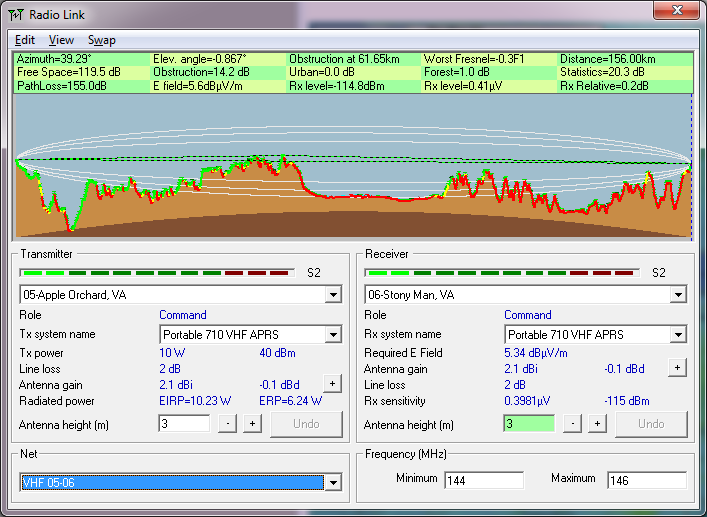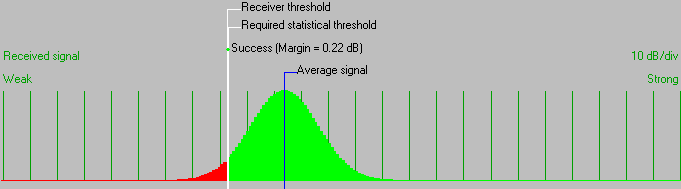• Distance between 05-Apple Orchard, VA and 06-Stony Man, VA is 156.0 km (96.9 miles)
• True North Azimuth = 39.29°, Magnetic North Azimuth = 48.10°, Elevation angle = -0.8668°
• Terrain elevation variation is 1078.2 m
• Propagation mode is diffraction, single obstruction, 0.3F1 at 61.7km
• Average frequency is 145.000 MHz
• Free Space = 119.5 dB, Obstruction = 14.2 dB, Urban = 0.0 dB, Forest = 1.0 dB, Statistics = 20.3 dB
• Total propagation loss is 155.0 dB (interference mode (optimistic))
• System gain from 05-Apple Orchard, VA to 06-Stony Man, VA is 155.2 dB
• System gain from 06-Stony Man, VA to 05-Apple Orchard, VA is 155.2 dB
• Worst reception corresponds to the required signal to meet 95.000% of situations